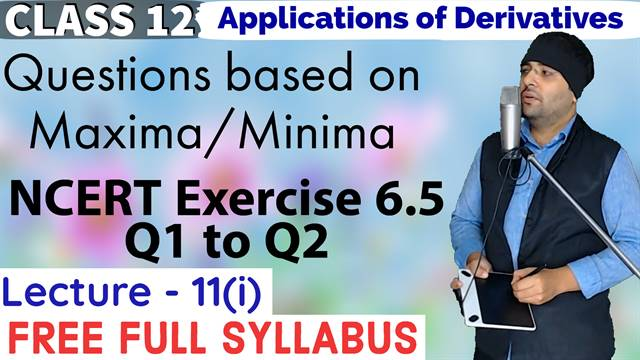# Exercise 6.5 (Q1 to Q2) Application of Derivatives Class 12 Maths Chapter 6## Topics/Questions discussed in this video:

Uses and difference between a function and an equation

Difference between maxima / minima and maximum / minimum.

How to study NCERT exercise 6.5 maxima and minima

Different types of maxima / minima or maximum / minimum (Global maximum, global minimum, absolute maximum, absolute minimum, local maximum, local minimum)

 NCERT EXERCISE 6.5

Question 1. Find the maximum and minimum values, if any, of the following functions given by
(i) f(x) = (2x - 1)^2 + 3
(ii) f(x) = 9x^2 + 12x + 2
(iii) f(x) = -(x - 1)^2 + 10
(iv) g(x) = x^3 + 1

Question 2. Find the maximum and minimum values, if any, of the following functions given by
(i) f(x) = |x + 2| - 1
(ii) g(x) = - |x + 1| + 3
(iii) h(x) = \sin (2x) + 5
(iv) f(x) = |\sin 4x + 3|
(v) h(x) = x + 1, x \in (-1, 1)

#### Categories## Agam Sir

Teacher

Ashish Kumar a.k.a Agam Sir has vast experience of 12+ years of teaching Mathematics and Physics. He has skills (academic and vocational) that go with working alongside people, especially young people, their parent(s) and the institutes that works with him. He always seek to be an agent of positive change and progression, a mentor/ trainer/ educator for learners/students, seeing that their needs are ideally met.